# Efficient spin filter using multi-terminal quantum dot with spin-orbit interaction

## Abstract

We propose a multi-terminal spin filter using a quantum dot with spin-orbit interaction. First, we formulate the spin Hall effect (SHE) in a quantum dot connected to three leads. We show that the SHE is significantly enhanced by the resonant tunneling if the level spacing in the quantum dot is smaller than the level broadening. We stress that the SHE is tunable by changing the tunnel coupling to the third lead. Next, we perform a numerical simulation for a multi-terminal spin filter using a quantum dot fabricated on semiconductor heterostructures. The spin filter shows an efficiency of more than 50% when the conditions for the enhanced SHE are satisfied.

PACS numbers: 72.25.Dc,71.70.Ej,73.63.Kv,85.75.-d

## Introduction

The injection and manipulation of electron spins in semiconductors are important issues for spin-based electronics, "spintronics." The spin-orbit (SO) interaction can be a key ingredient for both of them. The SO interaction for conduction electrons in direct-gap semiconductors is written as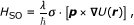(1)

where U(r) is an external potential, and σ indicates the electron spin s= σ/2. The coupling constant λ is largely enhanced in narrow-gap semiconductors such as InAs, compared with the value in the vacuum .

In two-dimensional electron gas (2DEG; xy plane) in semiconductor heterostructures, an electric field perpendicular to the 2DEG,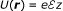, induces the Rashba SO interaction [3, 4]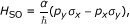(2)

where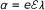. The Rashba SO interaction can be tuned by the external electric field, or the gate voltage . In the spin transistor proposed by Datta and Das , electron spins are injected into the 2DEG from a ferromagnet, and manipulated by tuning the strength of Rashba SO interaction. However, the spin injection from a ferromagnetic metal to semiconductors is generally not efficient, less than 0.1%, because of the conductivity mismatch . To overcome this difficulty, the SO interaction may be useful for the spin injection into semiconductor without ferromagnets. Several spin filters were proposed utilizing the SO interaction, e.g., three-terminal devices based on the spin Hall effect (SHE) , a triple-barrier tunnel diode , a quantum point contact [14, 15], and an open quantum dot .

The SHE is one of the phenomena utilized to create a spin current in the presence of SO interaction. There are two types of SHE. One is an intrinsic SHE which creates a dissipationless spin current in the perfect crystal . The other is an extrinsic SHE caused by the spin-dependent scattering of electrons by impurities . In our previous articles , we have formulated the extrinsic SHE in semiconductor heterostructures with an artificial potential created by antidot, scanning tunnel microscope (STM) tip, etc. The artificial potential is electrically tunable and may be attractive as well as repulsive. We showed that the SHE is significantly enhanced by the resonant scattering when the attractive potential is properly tuned. We proposed a multi-terminal spin filter including the artificial potential, which shows an efficiency of more than 50% .

In the present article, we investigate an enhancement of the SHE by the resonant tunneling through a quantum dot (QD) with strong SO interaction, e.g., InAs QD . The QD shows a peak structure of the current as a function of gate voltage, the so-called Coulomb oscillation. At the current peaks, the resonant tunneling takes place at low temperatures. First, we consider an impurity Anderson model with three leads, as shown in Figure 1a. There are two energy levels in the QD. We show a remarkable enhancement of the SHE when the level spacing in the QD is smaller than the level broadening. The SHE is electrically tunable by changing the tunnel coupling to the third lead.

Next, we perform a numerical simulation for a spin-filtering device fabricated on semiconductor heterostructures, in which a QD is connected to three leads (Figure 1b). The device is described using the tight-binding model of square lattice, which discretizes the two-dimensional space . We find that the spin filter indicates an efficiency of more than 50% when some conditions are satisfied.

## Formulation of spin Hall effect

To formulate the SHE in a multi-terminal QD, we begin with an impurity Anderson model shown in Figure 1a. The number of leads is denoted by N (N ≥ 2). As a minimal model, we consider two energy levels in the QD; ε 1, and ε 2. We assume that the wavefunctions, ψ 1 and ψ 2, in the QD are real in the absence of a magnetic field. Since the SO interaction (1) includes the momentum p= - , which is a pure imaginary operator, the diagonal elements of the SO interaction, 〈j|H SO|j〉 (j = 1, 2), disappear. The off-diagonal elements are denoted by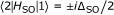for spin ±1/2 in the direction of 〈2|(p× U)|1〉.

The state |j〉 in the QD is connected to lead α by tunnel coupling, V α,j (j = 1, 2). The strength of the tunnel coupling is characterized by the level broadening, Γ α = πν α (V α,1 2 + V α,2 2), where ν α is the density of states in the lead. The leads have a single channel of conduction electrons. Unpolarized electrons are injected into the QD from source lead (α = S) and output to drain leads (Dn; n = 1, 2, , N - 1). The electric voltage is identical in the (N - 1) drain leads. The current to the drain Dn of each spin component, I n, is generally formulated in terms of Green functions in the QD .

We formulate the SHE in the vicinity of the Coulomb peaks where the resonant tunneling takes place. Neglecting the electron-electron interaction, we obtain an analytic expression of the conductance G nfor spin ±1/2 . We find that the SHE is absent (G1,+ = G1,-) when the number of leads is N = 2, as pointed out by other groups (see Ref.  and related references cited therein). For N = 3, the conductance to lead D1 is given by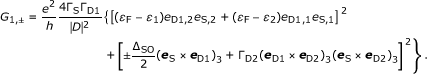(3)

Here, D is the determinant of the QD Green function, which is independent of spin ±1/2 (see Ref.  for detail). We introduce unit vectors, e α (α = S, D1, and D2), where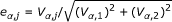, in the pseudo-spin space representing levels 1 and 2 in the QD. (a× b)3 = a 1 b 2 - a 2 b 1.

In Equation 3, the spin current [ (G n,+- G n,-)] stems from the interplay between SO interaction, ΔSO, and tunnel coupling to lead D2, ΓD2. We exclude specific situations in which two from e S, e D1, and e D2 are parallel to each other hereafter. We find the conditions for a large spin current as follows: (i) The level spacing, Δε = ε 2 - ε 1, is smaller than the level broadening by the tunnel coupling to leads S and D1, ΓS + ΓD1. (ii) The Fermi level in the leads is close to the energy levels in the QD (resonant condition). (iii) The level broadening by the tunnel coupling to lead D2, ΓD2, is comparable with the strength of SO interaction ΔSO.

Figure 2 shows the conductance of each spin, G 1,+ (solid line) and G 1,- (broken line), as a function of ε d = (ε 1 +ε 2)/2, in the case of N = 3. The conductance shows a peak reflecting the resonant tunneling around the Fermi level in the leads, which is set to be zero. We set ΓS = ΓD1 ≡ Γ, whereas (a) ΓD2 = 0.2Γ, (b) 0.5Γ, (c) Γ, and (d) 2Γ. The level spacing in the QD is Δε = 0.2Γ. The strength of SO interaction is ΔSO = 0.2Γ. The calculated results clearly indicate that the SHE is enhanced by the resonant tunneling around the peak. We obtain a large spin current when ΓD2 ~ ΔSO, as pointed out previously. Therefore, the SHE is tunable by changing the tunnel coupling to the third lead, ΓD2.

## Numerical simulation

To confirm the enhancement of SHE discussed using a simple model, we perform a numerical simulation for a spin-filtering device in which a QD is connected to three leads, as shown in Figure 1b. 2DEG in the xy plane is formed in a semiconductor heterostructure. Reservoir S is a source from which spin-unpolarized electrons are injected into the QD. The voltage is identical in reservoirs D1 and D2.

## Model

A QD is connected to reservoirs through quantum wires of width W. A hard-wall potential is assumed at the edges of the quantum wires. The QD is formed by quantum point contacts on the wires. The potential in a quantum wire along the x direction is given by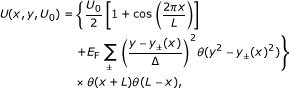(4)

with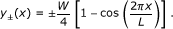(5)

where θ(t) is a step function [θ = 1 for t > 0, and θ = 0 for t < 0], U 0 is the potential height of the saddle point. The parameter Δ characterizes the confinement in the y direction, whereas L is the thickness of the potential barrier. When the electrostatic energy in the QD is changed by the gate voltage V g, the potential is modified to U(x, y, U 0 - eV g)+eV g inside the QD region [netted square region in Figure 1b] and U(x, y, U 0) outside of the QD region (The potential in the three quantum wires is overlapped by each other inside the QD region. Thus, we cut off the potential at the diagonal lines in the netted square region in Figure 1b).

The gradient of U gives rise to the SO interaction in Equation 1, as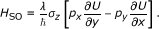(6)

Although the SO interaction is also created by the hard-wall potential at the edges of the leads, it is negligible because of a small amplitude of the wavefunction there .

The device is described using the tight-binding model of square lattice, which discretizes the real space in two dimensions [35, 38]. The width of the leads is W = 30a, with lattice constant a. The effective mass equation including the SO interaction in Equation 6 is solved numerically. The Hamiltonian is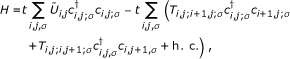(7)

where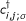and c i,j;σ are creation and annihilation operators of an electron, respectively, at site (i, j) with spin σ. t = ħ 2/(2m* a 2), and m* is the effective mass of electrons.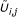is the potential energy at site (i, j), in units of t. The transfer term in the x direction is given by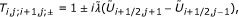(8)

whereas that in the y direction by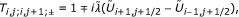(9)

with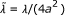.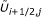is the potential energy at the middle point between the sites (i, j) and (i + 1, j), and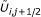is that of (i, j) and (i, j + 1).

We introduce a random potential w i,j in the QD region. -W ran/2 ≤ w i,j W ran/2. The randomness W ran is related to the mean free path Λ by the following equation :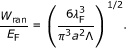(10)

We disregard the SO interaction induced by the random potential.

We assume that the Fermi wavelength is λ F = W/3 = 10a. The strength of SO interaction is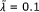, which corresponds to the value for InAs, λ = 117.1 Å2, with the width of the leads W = 30a ≈ 50 nm. The Fermi energy is given by E F/t = 2 - 2 cos(k F a), with k F = 2π/λ F. The thickness of tunnel barriers is L/λ F = 2. The randomness is W ran/E F = 1, which means that the mean free path is Λ/λF ≈ 19.4. The temperature is T = 0.

## Calculated results

Since the z component of spin is conserved with the SO interaction (6), we can evaluate the conductance for s z = ±1/2 separately. Using the Green's function and Landauer-Büttiker formula, we calculate the conductance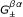from reservoir α to reservoir β, for spin s z = ±1/2 [35, 38, 39]. The total conductance is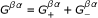, whereas the spin polarization in the z direction is given by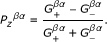(11)

We focus on the transport from reservoir S to D1 and omit the superscripts (β = D1, α = S) ofand P z βα .

Figure 3 presents the conductance G ± for spin s z = ±1/2 as a function of the gate voltage V g on the QD. We choose U S = U D1 = U D2 = 0.9E F for the tunnel barriers. The conductance G + (solid line) and G - (broken line) reflect the resonant tunneling through discrete energy levels formed in the QD region. Around some conductance peaks, e.g., at eV g/E F ≈ 0.13 and -0.03, the difference between G + and G - is remarkably enhanced. Thus, a large spin current is observed, which implies that two energy levels are close to each other around the Fermi level there.

The spin polarization P z is shown in Figure 4a for the range of 0.35 > eV g/E F > -0.25. Around the conductance peaks, a large spin polarization is observed. The efficiency of the spin filter becomes 37% at eV g/E F ≈ 0.13 and 42% at eV g/E F ≈ -0.03.

Next, we examine the tuning of the spin filter by changing the tunnel coupling to lead D2. In Figure 4, we set (b) U D2/E F = 0.8, (c) 0.7, and (d) 0.6 while both U S and U D1 are fixed at 0.9E F. As U D2 is decreased, the tunnel coupling becomes stronger. First, the spin polarization increases with an increase in the tunnel coupling. It is as large as 63% in the case of Figure 4b. With an increase in the tunnel coupling further, the spin polarization decreases (Figure 4c,d).

## Conclusions

We have formulated the SHE in a multi-terminal QD. The SHE is enhanced by the resonant tunneling through the QD when the level spacing is smaller than the level broadening. We have shown that the SHE is tunable by changing the tunnel coupling to the third lead. Next, the numerical simulation has been performed for a spin-filtering device using a multiterminal QD fabricated on semiconductor heterostructures. The efficiency of the spin filter can be larger than 50%.

## Abbreviations

QD:

quantum dot

STM:

scanning tunnel microscope

SHE:

spin Hall effect

SO:

spin-orbit.

## References

1. Žutić I, Fabian J, Das Sarma S: Spintronics: Fundamentals and applications. Rev Mod Phys 2004, 76: 323. 10.1103/RevModPhys.76.323

2. Winkler R: Spin-Orbit Coupling Effects in Two-Dimensional Electron and Hole Systems. Berlin Heidelberg: Springer; 2003.

3. Rashba EI: Properties of semiconductors with an extremum loop.1. Cyclotron and combinational resonance in a magnetic field perpendicular to the plane of the loop. Sov Phys Solid State 1960, 2: 1109.

4. Bychkov YuA, Rashba EI: Oscillatory effects and the magnetic susceptibility of carriers in inversion layers. J Phys C 1984, 17: 6039. 10.1088/0022-3719/17/33/015

5. Nitta J, Akazaki T, Takayanagi H, Enoki T: Gate Control of Spin-Orbit Interaction in an Inverted In 0.53 Ga 0.47 As/In 0.52 Al 0.48 As Heterostructure. Phys Rev Lett 1997, 78: 1335. 10.1103/PhysRevLett.78.1335

6. Grundler D: Large Rashba Splitting in InAs Quantum Wells due to Electron Wave Function Penetration into the Barrier Layers. Phys Rev Lett 2000, 84: 6074. 10.1103/PhysRevLett.84.6074

7. Sato Y, Kita T, Gozu S, Yamada S: Large spontaneous spin splitting in gate-controlled two-dimensional electron gases at normal In 0.75 Ga 0.25 As = In 0.75 Al 0.25 As heterojunctions. J Appl Phys 2001, 89: 8017. 10.1063/1.1362356

8. Datta S, Das B: Electronic analog of the electro-optic modulator. Appl Phys Lett 1990, 56: 665. 10.1063/1.102730

9. Schmidt G, Ferrand D, Molenkamp LW, Filip AT, van Wees BJ: Fundamental obstacle for electrical spin injection from a ferromagnetic metal into a diffusive semiconductor. Phys Rev B 2000, 62: R4790. 10.1103/PhysRevB.62.R4790

10. Kiselev AA, Kim KW: T-shaped ballistic spin filter. Appl Phys Lett 2001, 78: 775. 10.1063/1.1347023

11. Kiselev AA, Kim KW: T-shaped spin filter with a ring resonator. J Appl Phys 2003, 94: 4001. 10.1063/1.1601313

12. Yamamoto M, Kramer B: A three-terminal spin filter induced by spin-orbit interaction in the presence of an antidot. J Appl Phys 2008, 103: 123703. 10.1063/1.2938072

13. Koga T, Nitta J, Takayanagi H, Datta S: Spin-Filter Device Based on the Rashba Effect Using a Nonmagnetic Resonant Tunneling Diode. Phys Rev Lett 2002, 88: 126601.

14. Eto M, Hayashi T, Kurotani Y: Spin Polarization at Semiconductor Point Contacts in Absence of Magnetic Field. J Phys Soc Jpn 2005, 74: 1934. 10.1143/JPSJ.74.1934

15. Silvestrov PG, Mishchenko EG: Polarized electric current in semiclassical transport with spin-orbit interaction. Phys Rev B 2006, 74: 165301.

16. Sharma P, Brouwer PW: Mesoscopic Effects in Adiabatic Spin Pumping. Phys Rev Lett 2003, 91: 166801.

17. Bardarson JH, Adagideli I, Jacquod P: Mesoscopic Spin Hall Effect. Phys Rev Lett 2007, 98: 196601.

18. Krich JJ, Halperin BI: Spin-polarized current generation from quantum dots without magnetic fields. Phys Rev B 2008, 78: 035338.

19. Krich JJ: Fluctuations of spin transport through chaotic quantum dots with spin-orbit coupling. Phys Rev B 2009, 80: 245313.

20. Murakami S, Nagaosa N, Zhang S-C: Dissipationless Quantum Spin Current at Room Temperature. Science 2003, 301: 1348. 10.1126/science.1087128

21. Sinova J, Culcer D, Niu Q, Sinitsyn NA, Jungwirth T, MacDonald AH: Universal Intrinsic Spin Hall Effect. Phys Rev Lett 2004, 92: 126603.

22. Wunderlich J, Kaestner B, Sinova J, Jungwirth T: Experimental Observation of the Spin-Hall Effect in a Two-Dimensional Spin-Orbit Coupled Semiconductor System. Phys Rev Lett 2005, 94: 047204.

23. Dyakonov MI, Perel VI: Current-induced spin orientation of electrons in semiconductors. Phys Lett 1971, 35A: 459.

24. Engel HA, Halperin BI, Rashba EI: Theory of Spin Hall Conductivity in n-Doped GaAs. Phys Rev Lett 2005, 95: 166605.

25. Kato YK, Myers RC, Gossard AC, Awschalom DD: Observation of the Spin Hall Effect in Semiconductors. Science 2004, 306: 1910. 10.1126/science.1105514

26. Eto M, Yokoyama T: Enhanced Spin Hall Effect in Semiconductor Heterostructures with Artificial Potential. J Phys Soc Jpn 2009, 78: 073710. 10.1143/JPSJ.78.073710

27. Yokoyama T, Eto M: Enhanced spin Hall effect by tuning antidot potential: Proposal for a spin filter. Phys Rev B 2009, 80: 125311.

28. Yokoyama T, Eto M: Spin filter due to spin Hall effect with axially asymmetric potential. Physica E 2010, 42: 956. 10.1016/j.physe.2009.10.061

29. Igarashi Y, Jung M, Yamamoto M, Oiwa A, Machida T, Hirakawa K, Tarucha S: Spin-half Kondo effect in a single self-assembled InAs quantum dot with and without an applied magnetic field. Phys Rev B 2007, 76: 081303(R).

30. Fasth C, Fuhrer A, Samuelson L, Golovach VN, Loss D: Direct Measurement of the Spin-Orbit Interaction in a Two-Electron InAs Nanowire Quantum Dot. Phys Rev Lett 2007, 98: 266801(R).

31. Vachon M, Raymond S, Babinski A, Lapointe J, Wasilewski Z, Potemski M: Energy shell structure of a single InAs/GaAs quantum dot with a spin-orbit interaction. Phys Rev B 2009, 79: 165427.

32. Pfund A, Shorubalko I, Ensslin K, Leturcq R: Dynamics of coupled spins in quantum dots with strong spin-orbit interaction. Phys Rev B 2009, 79: 121306(R).

33. Takahashi S, Igarashi Y, Deacon RS, Oiwa A, Shibata K, Hirakawa K, Tarucha S: Quantitative evaluation of spin-orbit interaction in InAs quantum dots. J Phys Conf Ser 2009, 150: 022084. 10.1088/1742-6596/150/2/022084

34. Takahashi S, Deacon RS, Yoshida K, Oiwa A, Shibata K, Hirakawa K, Tokura Y, Tarucha S: Large Anisotropy of the Spin-Orbit Interaction in a Single InAs Self-Assembled Quantum Dot. Phys Rev Lett 2010, 104: 246801.

35. Datta S: Electronic Transport in Mesoscopic Systems. Cambridge: Cambridge University Press; 1995.

36. Meir Y, Wingreen NS: Landauer formula for the current through an interacting electron region. Phys Rev Lett 1992, 68: 2512. 10.1103/PhysRevLett.68.2512

37. Eto M, Yokoyama T: Quantum Dot Spin Filter in Resonant Tunneling and Kondo Regimes. J Phys Soc Jpn 2010, 79: 123711. 10.1143/JPSJ.79.123711

38. Ando T: Quantum point contacts in magnetic fields. Phys Rev B 1991, 44: 8017. 10.1103/PhysRevB.44.8017

39. Yamamoto M, Ohtsuki T, Kramer B: Spin polarization in a T-shaped conductor induced by strong Rashba spin-orbit coupling. Phys Rev B 2005, 72: 115321.

## Acknowledgements

This work was partly supported by a Grant-in-Aid for Scientific Research from the Japan Society for the Promotion of Science, and by Global COE Program "High-Level Global Cooperation for Leading-Edge Platform on Access Space (C12)." T. Y. is a Research Fellow of the Japan Society for the Promotion of Science.

## Author information

Authors

### Corresponding author

Correspondence to Tomohiro Yokoyama.

### Competing interests

The authors declare that they have no competing interests.

### Authors' contributions

TY participated the discussion of the analytical model and carried out the numerical calculation. ME carried out the analytical formulation of spin Hall effect. All authors conceived of the study, drafted the manuscript, read and approved the final manuscript.

## Authors’ original submitted files for images

Below are the links to the authors’ original submitted files for images.

## Rights and permissions

Open Access This article is distributed under the terms of the Creative Commons Attribution 2.0 International License (https://creativecommons.org/licenses/by/2.0), which permits unrestricted use, distribution, and reproduction in any medium, provided the original work is properly cited.

Reprints and Permissions

Yokoyama, T., Eto, M. Efficient spin filter using multi-terminal quantum dot with spin-orbit interaction. Nanoscale Res Lett 6, 436 (2011). https://doi.org/10.1186/1556-276X-6-436

• Accepted:

• Published:

• DOI: https://doi.org/10.1186/1556-276X-6-436

### Keywords

• Spin Polarization
• Quantum Wire
• Spin Current
• Resonant Tunneling
• Level Spacing